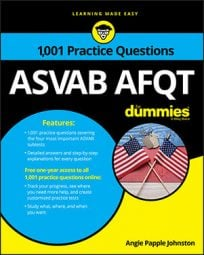##### ASVAB AFQTIf you're not familiar with slope-intercept form, then you will definitely want to learn it for the Mathematics Knowledge subtest on the ASVAB. The formula for slope-intercept form is y
= mx + b, where m represents slope and b represents the y-intercept.

## Practice questions

1. What is y + 3x – 6 = 0 in slope-intercept form?

A. y = 3x + 6 B. y = –3x + 6 C. y = –3x – 6 D. 6y = –3x

2. Rewrite in slope-intercept form:

x + y + 9 – 4x + 2y = 0

1. The correct answer is Choice (B).

The formula for slope-intercept form is y = mx + b, with m representing the slope and b representing the y-intercept. Isolate y:

2. The correct answer is Choice (D).

The formula for slope-intercept form is y = mx + b, so combine like terms and isolate y:

Simplify the equation by dividing each term by 3: